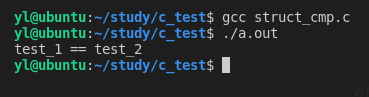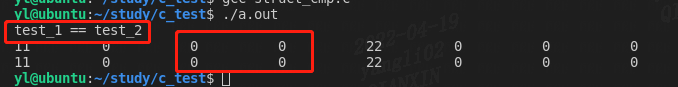# C语言是否能用memcmp函数比较结构体## 1.demo

``````#include <stdio.h>
#include <stdlib.h>
#include <string.h>

struct test{
short a;
int b;
};

int main()
{
struct test test_1 = {
.a = 1,
.b = 2,
};

struct test test_2 = {
.a = 1,
.b = 2,
};

if(memcmp(&test_1, &test_2, sizeof(struct test)) == 0){
printf("test_1 == test_2\n");
}else{
printf("test_1 != test_2\n");
}

return 0;
}``````

## 2.memcmp函数

``````#include <string.h>

int memcmp(const void *s1, const void *s2, size_t n);``````

## 3.测试分析

x86_64架构 对齐的原则：任何K字节的基本对象的地址必须是K的倍数。 比如：一个short对象的起始地址一定是2的倍数，一个int对象的起始地址一定是4的倍数。

``````#include <stdio.h>
#include <stdlib.h>
#include <string.h>

struct test{
short a;
int b;
};

int main()
{
struct test test_1 = {
.a = 11,
.b = 22,
};

struct test test_2 = {
.a = 11,
.b = 22,
};

char *p1 = (char *)&test_1;
for(int i = 0; i < sizeof(struct test); i++){
printf("%10d ", *p1);
p1++;
}
printf("\n");

char *p2 = (char *)&test_2;
for(int i = 0; i < sizeof(struct test); i++){
printf("%10d ", *p2);
p2++;
}

printf("\n");

return 0;
}``````

``````#include <stdio.h>
#include <stdlib.h>
#include <string.h>

struct test{
int a;
int b;
};

int main()
{
struct test test_1 = {
.a = 1,
.b = 2,
};

struct test test_2 = {
.a = 1,
.b = 2,
};

if(memcmp(&test_1, &test_2, sizeof(struct test)) == 0){
printf("test_1 == test_2\n");
}else{
printf("test_1 != test_2\n");
}

return 0;
}``````修改方案2： 将 struct test test_1 和 struct test test_2结构体改为全局变量：

``````#include <stdio.h>
#include <stdlib.h>
#include <string.h>

struct test{
short a;
int b;
};

struct test test_1 = {
.a = 11,
.b = 22,
};

struct test test_2 = {
.a = 11,
.b = 22,
};

int main()
{

if(memcmp(&test_1, &test_2, sizeof(struct test)) == 0){
printf("test_1 == test_2\n");
}else{
printf("test_1 != test_2\n");
}

char *p1 = (char *)&test_1;
for(int i = 0; i < sizeof(struct test); i++){
printf("%-10d ", *p1);
p1++;
}
printf("\n");

char *p2 = (char *)&test_2;
for(int i = 0; i < sizeof(struct test); i++){
printf("%-10d ", *p2);
p2++;
}

printf("\n");

return 0;
}``````当把 struct test test_1 和 struct test test_2结构体改为全局变量后，由于Linux c中规定未初始化的全局变量，运行时会将其初始化为0，所以填充的两个字节的值也是0，那么这种情况下就可以用 memcmp 比较了。

linux系统中：未初始化的全局变量属于可执行文件（ELF格式）的bss段，bss段不占用磁盘空间，仅仅是一个占位符，这样可以节省磁盘空间大小。在运行时，系统将bss段全部初始化为0。

## 参考资料

-- 展开阅读全文 --

BUUCTF Web [极客大挑战 2019]Knife
« 上一篇 06-24